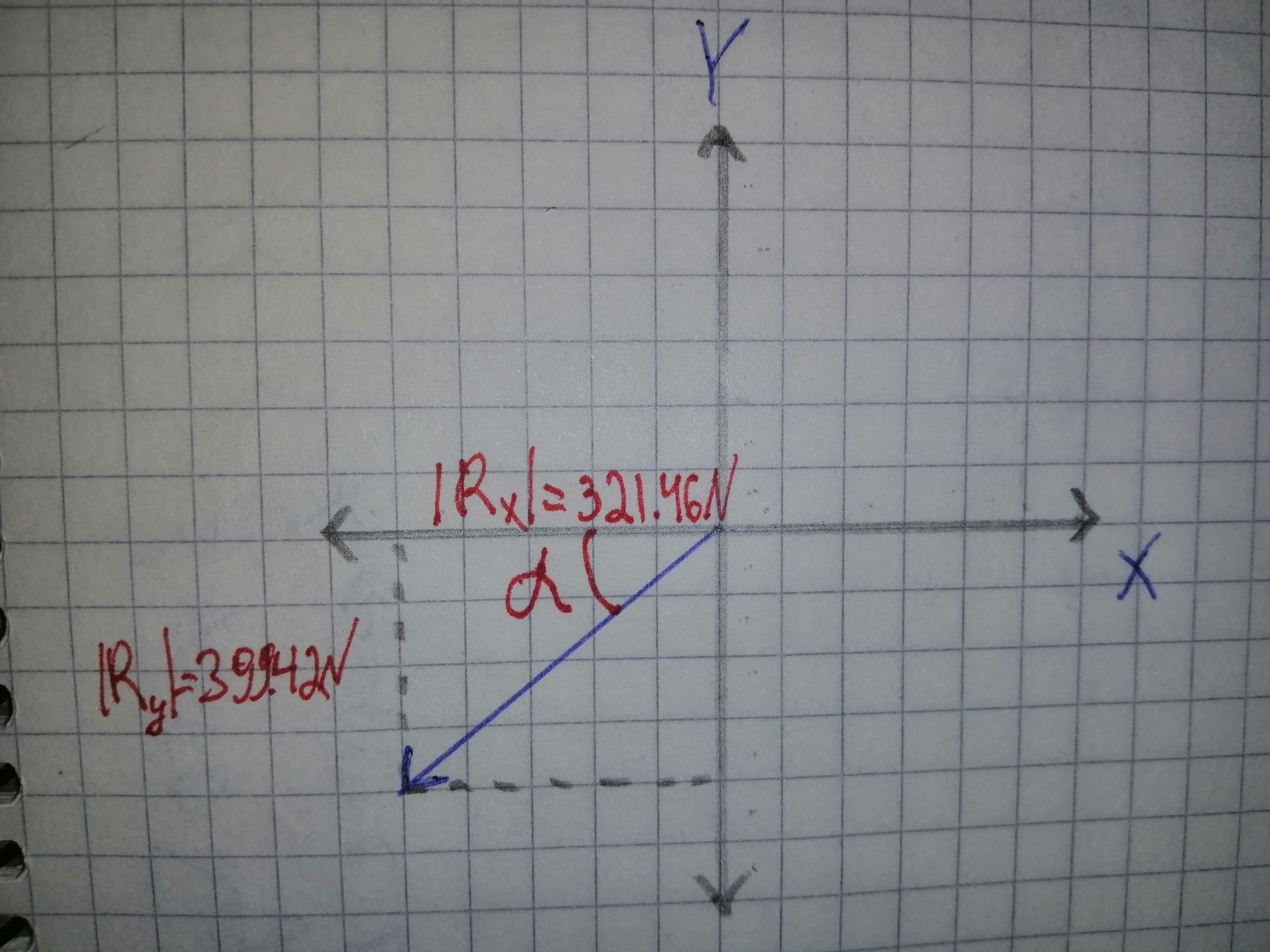# Find the sum of the given vectors displaystyle{700}{N}angle{320}^{circ}+{400}{N}angle{20}^{circ} Rx = ? Ry = ? R = ? theta = ?djeljenike 2021-02-06 Answered
Find the sum of the given vectors
$700N\mathrm{\angle }{320}^{\circ }+400N\mathrm{\angle }{20}^{\circ }$
$Rx=?$
$Ry=?$
$R=?$
$\theta =?$
You can still ask an expert for help

• Questions are typically answered in as fast as 30 minutes

Solve your problem for the price of one coffee

• Math expert for every subject
• Pay only if we can solve itJozlyn

Step 1The given expression is,
$700N\mathrm{\angle }{230}^{\circ }+400N\mathrm{\angle }{20}^{\circ }$
The angle of vector is measured whith positive x-asis.
Step 2
For
On resolving the vector, we get
${f}_{1}=700N\left[\left(-\mathrm{cos}\left({50}^{\circ }\right)\right)\stackrel{^}{i}+\left(-\mathrm{sin}\left({50}^{\circ }\right)\right)\stackrel{^}{j}\right]$
$=-\left(697.34N\right)\stackrel{^}{i}-\left(536.23N\right)j$
For on resolving we get
${F}_{2}=400N\left[\left(\mathrm{cos}\left({20}^{\circ }\right)\right)\stackrel{^}{i}+\left(\mathrm{sin}\left({20}^{\circ }\right)\right)\stackrel{^}{j}\right]$
$=\left(375.88N\right)\stackrel{^}{i}+\left(136.81N\right)\stackrel{^}{j}$
Step 3
$R={F}_{1}+{F}_{2}$
$=\left[-\left(697.34N\right)\stackrel{^}{i}-\left(536.23N\right)\stackrel{^}{j}\right]+\left[\left(375.88N\right)\stackrel{^}{i}+\left(136.81N\right)\stackrel{^}{i}+\left(136.81N\right)\stackrel{^}{j}\right]$
$=\left[\left(-697.34+375.88\right)N\right]\stackrel{^}{i}+\left[\left(-536.23+136.81\right)N\right]\stackrel{^}{j}$
$=\left(-321.46N\right)\stackrel{^}{i}+\left(-399.42N\right)\stackrel{^}{j}$
Hence,
${R}_{x}=-321$
${R}_{y}=-399$
$R=\sqrt{{R}_{x}^{2}+{R}_{y}^{2}}$
$=\sqrt{{\left(-321\right)}^{2}+{\left(-399\right)}^{2}}$
$=513$
Step 4$\mathrm{tan}\alpha =\frac{|{R}_{y}|}{|{R}_{x}|}$
$\alpha ={\mathrm{tan}}^{-1},\frac{|{R}_{y}|}{|{R}_{x}|}$
$={\mathrm{tan}}^{-1}\left(\frac{399.42}{321.46}\right)$
$=51$
Then angle made by resultant vector with positive x-axis is,
$\theta ={180}^{\circ }+\alpha$
$={180}^{\circ }+{51.17}^{\circ }$